# Calculus 1 : Local Minimum

## Example Questions

### Example Question #1 : How To Find Local Minimum By Graphing Differential Equations

For the equation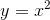, graph the function, and identify where the local minima is.

Minimum at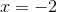.

Minimum at.

No minimum.

Minima atand.

Local minima atand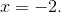Minimum at.

Explanation:

By graphing the equation, we can see that there minimum at, and that the graph continues to rise in both directions around this point, so this must be a local minimum. We also know that the graph rises infinitely in both directions, so this must be the only local minimum.

Another way to identify the local minima is by taking the derivative of the function and setting it equal to zero.

Using the power rule,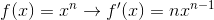we find the derivative to be,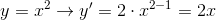.

From here we set the derivative equal to zero and solve for x. By doing this we will identify the critical values of the function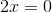Now we will plug in the x value and find the corresponding y value in the original equation. We will also plug in an x value that is lower than the critical x value and a x value that is higher than the critical value to confirm whether we have a local minima or maxima.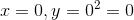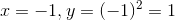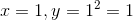Since both of the x values have a larger y value than the y value that corresponds to, we know that the minimum occurs at.

### Example Question #1 : How To Find Local Minimum By Graphing Differential Equations

Find the local minimum of the function.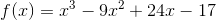None of theseExplanation:

The points where the derivative of the function is equal to 0 are called critical points. They are either local maximums, local minimums, or do not exist. The derivative of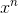is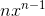. The derivative of the function is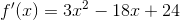.

We must now set it equal to zero and factor to solve.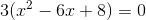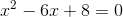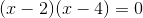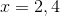Now we must plug in points to the left and right of the critical points into the derivative function to find the local min.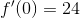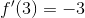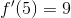This means the function is increasing until it hits x=2, then it decreases until it hits x=4 and begins increasing again. This makes x=4 the local minumum

### Example Question #3 : Graphing Differential Equations

Find thecoordinate of the local minumum of the following function.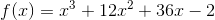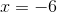None of these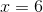Explanation:

The local maximums and minumums of a function are where the slope of the line tangent to the function is 0. To find the slope of the tangent line we must find the derivative. Then we must set ot equal to 0 and solve. The derivative ofis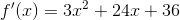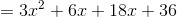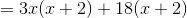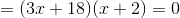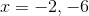The critical points are at the above two points. To find the minimum we must plug both back into the origianl function.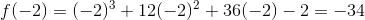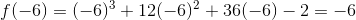Thus the local min is at x=-2.

### Example Question #4 : Graphing Differential Equations

You are given the function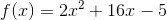. Find the minimum point of the function.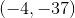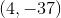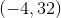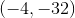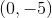Explanation:

To find the minimum of a function, start by finding the critical points of that function, or points where the derivative is equal to zero. Use the power rule to find the derivative: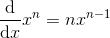Applying the power rule to the given equation, noting the constants in the first and second terms: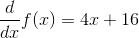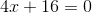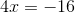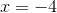Then check to see if the critical point is a maximum, minimum, or an inflection point by taking the second derivative, using the power rule once again.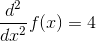Because the second derivative is positive, the critical pointis a minimum.

To find the point where the minimum occurs, plugback into the original equation and solve for.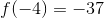Therefore, the minimum is### Example Question #1 : How To Find Local Minimum By Graphing Differential Equations

A functionis given by the equation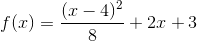.

By graphing the derivative of, whichvalue corresponds to the local minumum?Explanation:

The local minimum of a function can be found by finding the derivative and graphing it. The point in which the x axis is crossed from below gives the x position where the local minimum is found. Taking the derivative: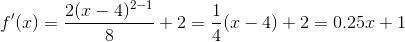The graph of the derivative is shown below: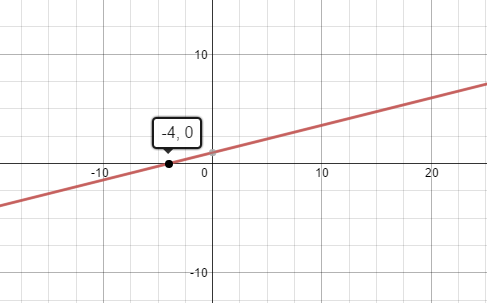As shown by the graph, the local minimum is found at x = -4.

### All Calculus 1 Resources